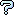All about flooble | fun stuff | Get a free chatterbox | Free JavaScript | Avatarsperplexus dot infoSumming Powers 2 (Posted on 2007-08-25)What is x + y + z if the following are true:

x4 + y4 + z4 = 11
x3 + y3 + z3 = 7
x2 + y2 + z2 = 3

Just think of this problem as the reverse of this one.

 Submitted by Brian Smith Rating: 4.0000 (4 votes) Solution: (Hide) The following identity shows the relationship between the sum and the sums of powers: 6*(x^4+y^4+z^4) = (x+y+z)^4 + 3*(x^2+y^2+z^2)^2 - 6*(x^2+y^2+z^2)(x+y+z)^2 + 8*(x^3+y^3+z^3)(x+y+z) Let x + y + z = N and substitute: 6*11 = N^4 + 3*3^2 - 6*3*N^2 + 8*7*N 0 = N^4 - 18*N^2 + 56*N - 39 N=1, -5.4414, 2.2207 +/- 1.4953*iComments: ( You must be logged in to post comments.)
 Subject Author Datere: Unreal! Ady TZIDON 2018-03-05 05:46:46re: solution K Sengupta 2008-07-16 11:47:01solution Jason Scott Clark 2007-08-26 12:51:03Solution K Sengupta 2007-08-25 23:49:20Unreal! Steve Herman 2007-08-25 21:19:16Please log in:

 Search: Search body:
Forums (0)